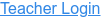### Description

Explore vectors in 1D or 2D, and discover how vectors add together. Specify vectors in Cartesian or polar coordinates, and see the magnitude, angle, and components of each vector. Experiment with vector equations and compare vector sums and differences.

### Sample Learning Goals

• Describe a vector in your own words
• Explain a method to add vectors
• Compare and contrast the component styles
• Decompose a vector into components
• Describe what happens to a vector when it is multiplied by a scalar
• Arrange vectors graphically to represent vector addition or subtraction
Version 1.0.0